# What’s the (hi)story of my network?

First an announcement: we have researcher and postdoc positions available in Theory Group at MSR, and I may have a position for an intern in the summer. If you are a talented young mathematician with interests in the kind of things I blog about please get in touch with me by email for more details about these positions.

The topic of this post is the reason why the blog has been silent for the past 3 months: I have just uploaded three new network papers, written with amazing co-authors (Luc Devroye, Jian Ding, Ronen Eldan, Gabor Lugosi, Elchanan Mossel, and Miklos Racz), and which can be found here, here, and here. The overall goal of these works is to realize (a version of) the vision that Nati Linial presented in a public lecture at the Simons Institute in Berkeley about a year ago. As Nati puts it, there is a need for a statistical theory on graphs, and specifically he writes that we should “Develop a battery of generative models of graphs and methods to recover the appropriate model from the input graph”. Obviously this type of question has already been looked at by statisticians, but somehow I feel like they have been shy in the models and questions that they consider. I think that there is a huge opportunity to look at more exotic (yet practically relevant) estimation problems with network data. To simplify, and get at the heart of the matter, I will essentially discuss testing problems rather than estimation.

Is there geometry in my network?

In the space of testing problems on random graphs, the king of the hill is testing for community structure. A precise hypothesis testing problem considered by Ery Arias-Castro and Nicolas Verzelen (see here and here) is the following: Under the null hypothesis, the observed graphon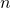vertices has been generated by the standard Erdos-Renyi random graph, where each edge appears independently with probability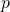. Under the alternative,comes from the modelwhich is similar toexcept that there is a subset ofvertices (the community) chosen uniformly at random and such that the connection probability between two vertices from that subset is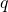instead of. The case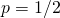and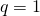corresponds to the now famous Planted Clique (PC) problem. In PC it is easy to see that the information theoretic limit for testability is whenis of order(use for instance this), but surprisingly we do not know any polynomial-time test when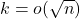. Bridging this gap is a major open problem.

Testing for community structure is of course very important in practice (and it’s also a beautiful mathematical question), but it seems a bit silly to restrict all the attention to this single question. In my joint work with Jian, Ronen and Miki we propose to test for geometric structure. This is also quite important in practice, as learning representations for the vertices of a graph is a fundamental primitive to many network analysis techniques. The abstract of our paper reads as follows, and I refer you to the paper itself for more details:

We study the problem of detecting the presence of an underlying high-dimensional geometric structure in a random graph. Under the null hypothesis, the observed graph is a realization of an Erdos-Renyi random graph. Under the alternative, the graph is generated from the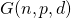model, where each vertex corresponds to a latent independent random vector uniformly distributed on the sphere, and two vertices are connected if the corresponding latent vectors are close enough. In the dense regime (i.e.,is a constant), we propose a near-optimal and computationally efficient testing procedure based on a new quantity which we call signed triangles. The proof of the detection lower bound is based on a new bound on the total variation distance between a Wishart matrix and an appropriately normalized GOE matrix. In the sparse regime, we make a conjecture for the optimal detection boundary. We conclude the paper with some preliminary steps on the problem of estimating the dimension in.

Where did my network come from?

The two other papers (1, 2) are follow-ups on the project that I described a few months ago in this blog post. In a nutshell the goal was to understand if the Preferential Attachment model depends on its initial condition. See for example these beautiful pictures by Igor Kortchemski of the Preferential Attachment tree on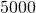vertices with different seeds: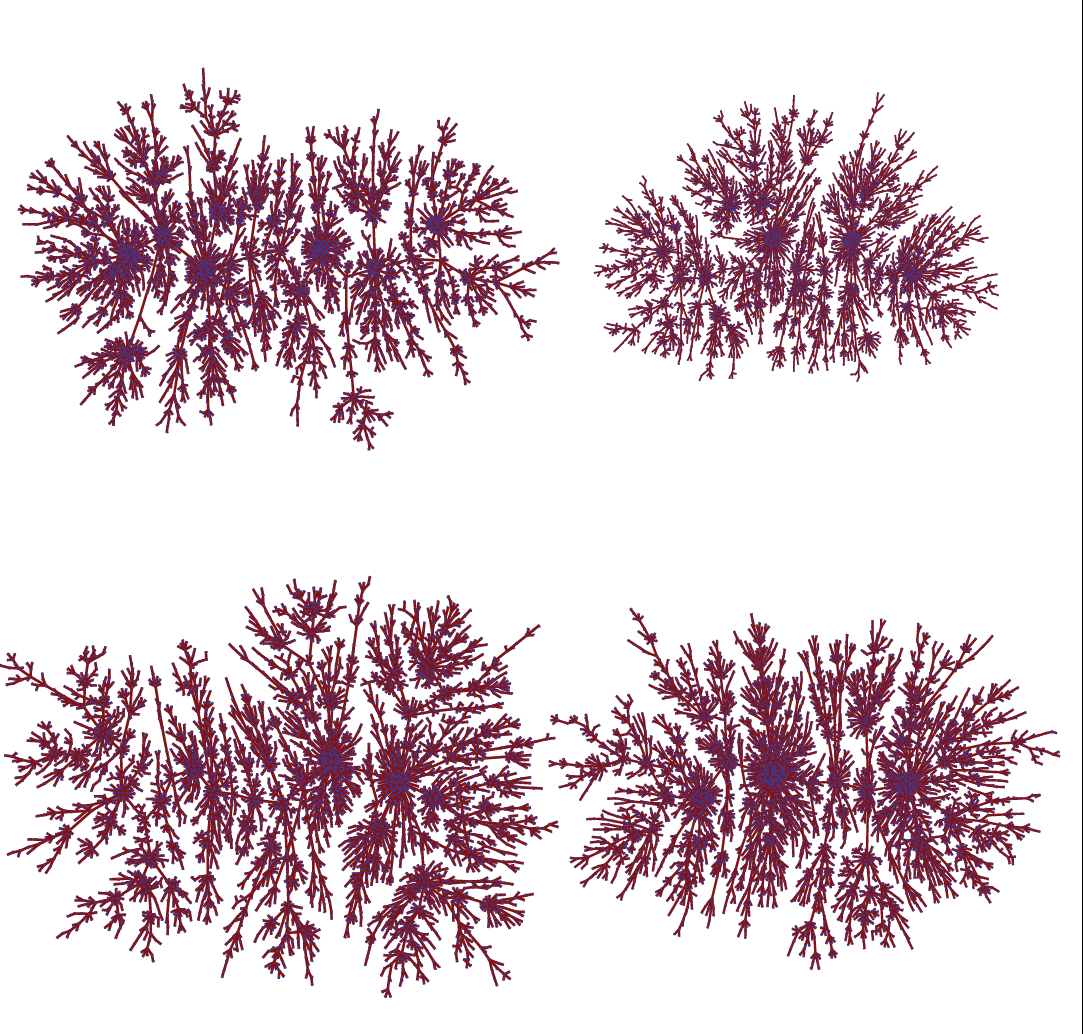(click here to see the seeds for the above pictures, and see here and here for pictures with other seeds). With Elchanan and Miki we conjectured that any initial condition leads to a different model, and we were able to partially prove this conjecture. The full conjecture was proved in a beautiful follow-up paper by a group of fellow French, Nicolas Curien, Thomas Duquesne, Igor Kortchemski, and Ioan Manolescu. Using their new proof technique (but with a different statistic) we (Ronen, Elchanan, Miki, and I) were then able to extend the theorem to the Uniform Attachment model, in which case the result is perhaps even more surprising (in fact I was originally convinced that the seed could not possibly have an influence in the Uniform Attachment model!). The paper can be found here.

The Seed Theorem emerging from these works opens up the problem of actually finding the seed. This is the problem we looked at with Luc and Gabor (in the special case where the seed is a single vertex), and the abstract of the paper reads as follows:

We investigate algorithms to find the first vertex in large trees generated by either the uniform attachment or preferential attachment model. We require the algorithm to output a set ofvertices, such that, with probability at least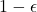, the first vertex is in this set. We show that for any, there exist such algorithms withindependent of the size of the input tree. Moreover, we provide almost tight bounds for the best value ofas a function of. In the uniform attachment case we show that the optimalis subpolynomial in, and that it has to be at least superpolylogarithmic. On the other hand, the preferential attachment case is exponentially harder, as we prove that the bestis polynomial in. We conclude the paper with several open problems.

Open problems

The four papers (1, 2, 3, 4) contain about a dozen open problems, and we could have easily extended the list to twenty open problems (for example I have added a few open problems in my talk at Oberwolfach two weeks ago, see here for Louigi Addario-Berry‘s notes). Can you solve one of them? Or even better, can you propose new interesting graph hypothesis testing problems?

This entry was posted in Random graphs. Bookmark the permalink.

## 3 Responses to "What’s the (hi)story of my network?"

•••